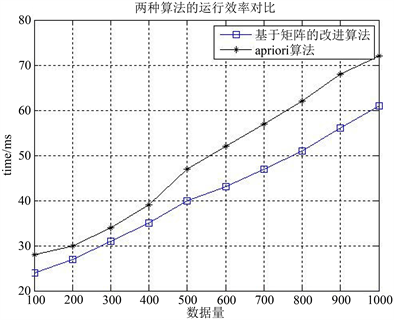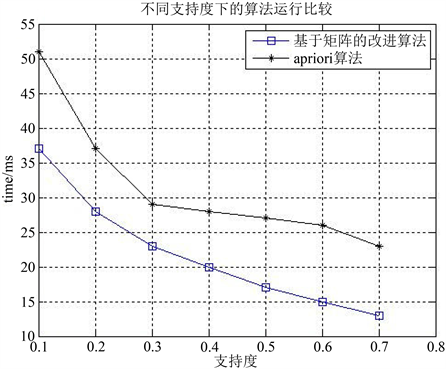﻿ 基于矩阵改进的关联规则算法研究

# 基于矩阵改进的关联规则算法研究Research on Improved Association Rules Algorithm Base on Matrix

Abstract: Apriori algorithm is a classical quantitative association rule algorithm. Traditional Apriori algo-rithms have many shortcomings in the computational power of large amounts of data and need to scan the database many times. An improved Apriori algorithm based on compressed matrix re-duced a large number of candidate items and the memory space of data. Experimental results showed that the improved algorithm can mine frequent items effectively and be less spaced than the classical Apriori algorithm.

Abstract:

1. 引言

2. 关联规则的基本概念

$\mathrm{support}\left(X\to Y\right)=P\left(XY\right)=\frac{|XY|}{|D|}$

$\text{confidence}\left(X\to Y\right)=P\left(Y|X\right)=\frac{|XY|}{|X|}$

3. Apriori的基本步骤

Apriori算法的主要步骤：

1) 先对事务数据库进行全部扫描。进行每个项集的比对，若满足关联规则的条件，则从事务数据库中生成频繁1-项集 ${L}_{1}$

2) 频繁项集的连接。由于算法是通过迭代递推的方式进行项集与项集之间的连接。当 $k\ge 1$ 时，频繁项集 ${L}_{k}$ 通过自身的连接来产生 $k+1$ 候选集 ${C}_{k+1}$

3) 候选集进行剪枝，删除不适合条件的项集。在频繁项集产生的候选集 ${C}_{k+1}$ 中，筛选满足条件的项集即项集出现的频度必须大于设定的最小支持度，删除不满足条件的项目集。

4) 生关联规则。由于规则算法原理是遍历整个数据库循环迭代计算频繁项集的基础上，所以产生的规则都是满足最小支持度的，然后得出项集的强关联规则。

4. 基于矩阵改进的Apriori算法

4.1. 关概念与性质

$D=\left[\begin{array}{cccc}{d}_{11}& {d}_{12}& \cdots & {d}_{1n}\\ {d}_{21}& {d}_{22}& \cdots & {d}_{2n}\\ ⋮& ⋮& \cdots & ⋮\\ {d}_{m1}& {d}_{\left(m2\right)}& \cdots & {d}_{mn}\end{array}\right]$

$\text{support}_\text{count}\left({I}_{j}\right)=\underset{i-1}{\overset{m}{\sum }}{d}_{ij}$

$\text{support}_\text{count}\left({I}_{1}\right)={d}_{11}+{d}_{21}+\cdots +{d}_{m1}$

${D}_{ij}={D}_{i}\wedge {D}_{j}=\left[\begin{array}{c}{d}_{1i}\wedge {d}_{1j}\\ {d}_{2i}\wedge {d}_{2j}\\ ⋮\\ {d}_{mi}\wedge {d}_{nj}\end{array}\right]$

$\text{support}_\text{count}\left({I}_{i}{I}_{j}\right)=\underset{k-1}{\overset{n}{\sum }}{d}_{{k}_{i}}\wedge {d}_{kj}$

$\text{support}_\text{count}\left({I}_{1}{I}_{2}\cdots {I}_{j}\right)=\underset{k-1}{\overset{n}{\sum }}\left(\left({d}_{{p}_{1}}\wedge {d}_{p2}\wedge \cdots \wedge {d}_{\left(pk-1\right)}\right)\wedge {d}_{pk}\right)$

4.2. 改进算法的主要步骤

1) 对事务数据库进行全部扫描。同时设定项集的支持度和置信度，若满足上述的条件，矩阵的行向量对应事务集，列为项集。为了方便矩阵计算，在项集布尔矩阵计算时增加 $sum_r$ 行用来计算每一列的和即求矩阵每一列对应项集的支持度。

2) 项集的布尔矩阵计算每列1的个数。增加矩阵的一行 $sum_r$ 用来统计每列1的数值，若该数值小于支持度，则把该值对应的列向量删除。其余的都是频繁1-项集对应的列向量，得频繁1-项集矩阵 $D\left(1\right)$，求得 ${L}_{1}$ 频繁项集。

3) 求 $k\left(k\ge 2\right)$ 维频繁项集的，对频繁 $D\left(k-1\right)$ 矩阵的对列向量“与”运算，在对运算之后的列向量求和，不符合条件的删除，剩下的列向量对应的是频繁项集，得频繁 $k-$ 项集矩阵 $D\left(k\right)$

4) 复执行上述的2和3步骤，经操作后把不满足的列向量不断删除，矩阵不断地被压缩。根据性质2，若频繁项集计算到 $\left(k-1\right)$ 项集的个数，即矩阵的列的个数小于k时，停止算法运算。

4.3. 应用实例

I：对事务表映射成布尔矩阵，事务包含该项目的值为1，否则为0。转换后的数据库如下表：

$\begin{array}{l}\text{ }\text{ }\text{ }\text{ }\text{ }\text{ }\text{ }\text{ }\text{\hspace{0.17em}}\text{\hspace{0.17em}}\text{\hspace{0.17em}}{I}_{1}\text{\hspace{0.17em}}\text{}\text{\hspace{0.17em}}{I}_{2}\text{\hspace{0.17em}}\text{\hspace{0.17em}}\text{}{I}_{3}\text{\hspace{0.17em}}\text{\hspace{0.17em}}{I}_{4}\text{\hspace{0.17em}}{I}_{5}\text{\hspace{0.17em}}\text{\hspace{0.17em}}\text{}{I}_{6}\text{\hspace{0.17em}}\text{}{I}_{7}\\ D=\left({D}_{1},{D}_{2},\cdots ,{D}_{7}\right)=\left[\begin{array}{ccccccc}1& 0& 1& 1& 0& 1& 0\\ 0& 1& 1& 1& 0& 1& 0\\ 0& 0& 0& 1& 1& 1& 0\\ 1& 1& 0& 0& 1& 0& 0\\ 1& 1& 1& 0& 0& 0& 0\\ 0& 0& 1& 0& 1& 0& 1\end{array}\right]\\ \text{ }\text{ }\text{ }\text{ }\text{ }\text{ }\text{ }\text{ }\text{\hspace{0.17em}}\text{\hspace{0.17em}}\text{\hspace{0.17em}}\left[3\text{\hspace{0.17em}}\text{}\text{\hspace{0.17em}}3\text{\hspace{0.17em}}\text{}\text{\hspace{0.17em}}4\text{\hspace{0.17em}}\text{}\text{\hspace{0.17em}}3\text{\hspace{0.17em}}\text{}\text{\hspace{0.17em}}3\text{\hspace{0.17em}}\text{}\text{\hspace{0.17em}}3\text{\hspace{0.17em}}\text{}\text{\hspace{0.17em}}\text{\hspace{0.17em}}1\right]\end{array}$

$\text{support}_\text{count}\left({I}_{1}\right)=\underset{i-1}{\overset{6}{\sum }}{d}_{i1}=3$

$\begin{array}{l}\text{ }\text{ }\text{ }{I}_{1}\text{\hspace{0.17em}}\text{}\text{\hspace{0.17em}}{I}_{2}\text{\hspace{0.17em}}\text{\hspace{0.17em}}\text{}{I}_{3}\text{\hspace{0.17em}}\text{\hspace{0.17em}}{I}_{4}\text{\hspace{0.17em}}{I}_{5}\text{\hspace{0.17em}}\text{\hspace{0.17em}}\text{}{I}_{6}\\ D\left(1\right)=\left[\begin{array}{cccccc}1& 0& 1& 1& 0& 1\\ 0& 1& 1& 1& 0& 1\\ 0& 0& 0& 1& 1& 1\\ 1& 1& 0& 0& 1& 0\\ 1& 1& 1& 0& 0& 0\\ 0& 0& 1& 0& 1& 0\end{array}\right]\end{array}$

II：生成频繁2-项集。由定义2，在求频繁2-项集时，利用经过逻辑“与”运算得2-项D(1)集矩阵，再对每一列进行计数，若列向量求和小于支持度的删除不满足的列向量得到频繁2-项集矩阵，则I12的支持度计数为：

${D}_{12}={D}_{1}\wedge {D}_{2}=\left[\begin{array}{c}{d}_{11}\wedge {d}_{12}\\ {d}_{21}\wedge {d}_{22}\\ ⋮\\ {d}_{61}\wedge {d}_{62}\end{array}\right]={\left(\begin{array}{cccccc}0& 0& 0& 1& 1& 0\end{array}\right)}^{T}$

$\begin{array}{l}\text{ }\text{ }\text{ }\text{\hspace{0.17em}}{I}_{12}\text{\hspace{0.17em}}{I}_{13}\text{\hspace{0.17em}}{I}_{23}\text{\hspace{0.17em}}{I}_{34}\text{\hspace{0.17em}}\text{\hspace{0.17em}}{I}_{36}\text{\hspace{0.17em}}\text{\hspace{0.17em}}{I}_{46}\\ D\left(2\right)=\left[\begin{array}{cccccc}0& 1& 0& 1& 1& 1\\ 0& 0& 1& 1& 1& 1\\ 0& 0& 0& 0& 0& 1\\ 1& 0& 0& 0& 0& 0\\ 1& 1& 1& 0& 0& 0\\ 0& 0& 0& 0& 0& 0\end{array}\right]\end{array}$

III：生成频繁3-项集。同理进行对上述相同的运算，根据性质1，频繁k-项集的事务个数的长度小于或等于k，若在生成频繁k项集，项集连接产生的事务个数大于k，则删除该项集的连接。若产生的项集为重复的项集，保留一个项集的列向量，其余删除，得频繁3-项集矩阵。

$\begin{array}{l}\text{ }\text{ }\text{\hspace{0.17em}}\text{\hspace{0.17em}}\text{\hspace{0.17em}}{I}_{346}\\ D\left(3\right)=\left[\begin{array}{c}1\\ 1\\ 0\\ 0\\ 0\\ 0\end{array}\right]\end{array}$

$\text{count}\left({I}_{346}\right)=2$

L3的项集的列向量只有1列，即个数只有1个，根据步骤(4)，小于4，则不用继续求L4。L3频繁项集I346为最大的频繁项集。

4.4. 算法实验与分析Figure 1. Running time under different amounts of dataFigure 2. Running time under different support

5. 结束语

NOTES

*通讯作者。

 简玉姣. 基于矩阵的相关规则挖掘算法研究[D]: [硕士学位论文]. 兰州: 兰州大学, 2013.

 Zhang, Y. and Chen, J. (2010) AVI: Based on the Vertical and Intersection Operation of the Improved Apriori Algorithm. Proceedings of the 2nd International Conference on Future Computer and Communication, Wuhan, 21-24 May 2010, V2-718-V2-721.
https://doi.org/10.1109/ICFCC.2010.5497596

 Wang, G.-F., Yu, X. Peng, D.-B., Cui, Y.-H. and Li, Q.-M. (2010) Research of Data Mining Based on Apriori Algorithm in Cutting Database. Proceedings of the 2010 International Conference on Mechanic Automation and Control Engineering, Wuhan, 26-28 Jun 2010, 3765-3768.

 付沙, 周航军. 关联规则挖掘Apriori算法的研究与改进[J]. 微电子学与计算机, 2013, 30(9): 110-114.

 赵志刚, 万军, 王芳. 一种基于向量的概率加权关联规则挖掘算法[J]. 计算机工程与科学, 2014, 36(2): 354-358.

 Vaithiyanathan, V., Rajeswari, K., Phalnikar, R. and Tonge, S. (2012) Improved Apriori Algorithm Based on Selection Criterion. Proceedings of the 2012 IEEE International Conference on Computational Intelligence & Computing Research, Coimbatore, 18-20 December 2012, 1-4.
https://doi.org/10.1109/ICCIC.2012.6510229

 Chen, Z., Cai, S.-B., Song, Q.-L. and Zhu, C.-L. (2011) An Improved Apriori Algorithm Based on Pruning Optimization and Transaction Reduction. Proceedings of the 2nd In-ternational Conference on Artificial Intelligence, Management Science and Electronic Commerce, Zhengzhou, 8-10 August 2011, 1908-1911.

Top